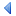UpTOC Index << 7 Included Tools < 8.16 Sequence Manipulation Up: 8 API Reference 8.18 Sorting > 9 Release Notes >>

## 8.17 Serialization of Euphoria Objects

### 8.17.1 Routines

#### 8.17.1.1 deserialize

```include std/serialize.e
namespace serialize
public function deserialize(object sdata, integer pos = 1)
```

Convert a serialized object in to a standard Euphoria object.

##### Parameters:
1. sdata : either a sequence containing one or more concatenated serialized objects or an open file handle. If this is a file handle, the current position in the file is assumed to be at a serialized object in the file.
2. pos : optional index into sdata. If omitted 1 is assumed. The index must point to the start of a serialized object.
##### Returns:

The return value, depends on the input type.

• If sdata is a file handle then this function returns a Euphoria object that had been stored in the file, and moves the current file to the first byte after the stored object.
• If sdata is a sequence then this returns a two-element sequence. The first element is the Euphoria object that corresponds to the serialized object that begins at index pos, and the second element is the index position in the input parameter just after the serialized object.

A serialized object is one that has been returned from the serialize function.

##### Example 1:
```sequence objcache
objcache = serialize(FirstName) &
serialize(LastName) &
serialize(PhoneNumber) &

sequence res
integer pos = 1
res = deserialize( objcache , pos)
FirstName = res pos = res
res = deserialize( objcache , pos)
LastName = res pos = res
res = deserialize( objcache , pos)
PhoneNumber = res pos = res
res = deserialize( objcache , pos)
Address = res pos = res
```
##### Example 2:
```sequence objcache
objcache = serialize({FirstName,
LastName,
PhoneNumber,

sequence res
res = deserialize( objcache )
FirstName = res
LastName = res
PhoneNumber = res
```
##### Example 3:
```integer fh
fh = open("cust.dat", "wb")
puts(fh, serialize(FirstName))
puts(fh, serialize(LastName))
puts(fh, serialize(PhoneNumber))
close(fh)

fh = open("cust.dat", "rb")
FirstName = deserialize(fh)
LastName = deserialize(fh)
PhoneNumber = deserialize(fh)
close(fh)
```
##### Example 4:
```integer fh
fh = open("cust.dat", "wb")
puts(fh, serialize({FirstName,
LastName,
PhoneNumber,
close(fh)

sequence res
fh = open("cust.dat", "rb")
res = deserialize(fh)
close(fh)
FirstName = res
LastName = res
PhoneNumber = res
```

#### 8.17.1.2 serialize

```include std/serialize.e
namespace serialize
public function serialize(object x)
```

Convert a standard Euphoria object in to a serialized version of it.

##### Parameters:
1. euobj : any Euphoria object.
##### Returns:

A sequence, this is the serialized version of the input object.

A serialized object is one that has been converted to a set of byte values. This can then by written directly out to a file for storage.

You can use the deserialize function to convert it back into a standard Euphoria object.

##### Example 1:
```integer fh
fh = open("cust.dat", "wb")
puts(fh, serialize(FirstName))
puts(fh, serialize(LastName))
puts(fh, serialize(PhoneNumber))
close(fh)

fh = open("cust.dat", "rb")
FirstName = deserialize(fh)
LastName = deserialize(fh)
PhoneNumber = deserialize(fh)
close(fh)
```
##### Example 2:
```integer fh
fh = open("cust.dat", "wb")
puts(fh, serialize({FirstName,
LastName,
PhoneNumber,
close(fh)

sequence res
fh = open("cust.dat", "rb")
res = deserialize(fh)
close(fh)
FirstName = res
LastName = res
PhoneNumber = res
```

#### 8.17.1.3 dump

```include std/serialize.e
namespace serialize
public function dump(sequence data, sequence filename)
```

Saves a Euphoria object to disk in a binary format.

##### Parameters:
1. data : any Euphoria object.
2. filename : the name of the file to save it to.
##### Returns:

An integer, 0 if the function fails, otherwise the number of bytes in the created file.

If the named file doesn't exist it is created, otherwise it is overwritten.

You can use the load function to recover the data from the file.

##### Example :
```include std/serialize.e
integer size = dump(myData, theFileName)
if size = 0 then
puts(1, "Failed to save data to file\n")
else
printf(1, "Saved file is %d bytes long\n", size)
end if
```

```include std/serialize.e
namespace serialize
```

Restores a Euphoria object that has been saved to disk by dump.

##### Parameters:
1. filename : the name of the file to restore it from.
##### Returns:

A sequence, the first element is the result code. If the result code is 0 then it means that the function failed, otherwise the restored data is in the second element.

This is used to load back data from a file created by the dump function.

##### Example :
```include std/serialize.eUpTOC Index << 7 Included Tools < 8.16 Sequence Manipulation Up: 8 API Reference 8.18 Sorting > 9 Release Notes >>